Updating search results...

# 6 Results

View
Selected filters:
• equations-and-expressions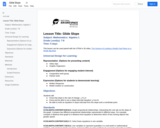Conditional Remix & Share Permitted
CC BY-NC
Rating
0.0 stars

Students explore slope calculations by calculating glide slope of planes landing on aircraft carriers.

Subject:
Mathematics
Material Type:
Lesson Plan
Provider:
National Air and Space Museum
Author:
National Air and Space Museum
06/13/2022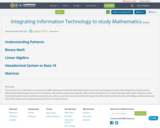Conditional Remix & Share Permitted
CC BY-SA
Rating
0.0 stars

This course is is a collection of resources on OER Commons curated for Adult Education instructors and students to show the integration of math into the Information Technology Career Sector. Students will analyze and practice specific skills related to being in IT as well as develop math skills. Modules in this curriculum guide can be studied in any particular order as one does not necessarily build upon the other. Each includes the idea of building mathematical and logic skills required for programming and other IT related careers.

Subject:
Career and Technical Education
Mathematics
Material Type:
Teaching/Learning Strategy
Unit of Study
05/30/2018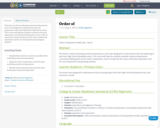Unrestricted Use
CC BY
Rating
0.0 stars

This lesson is about evaluating numerical expressions, and it was designed for adult learners who are preparing to take their High School Equivalency tests. This course will help the students evaluate numerical expressions correctly by following the correct order of operations, which includes the four basic arithmetical operations and the use of exponents and grouping symbols.

Subject:
Mathematics
Material Type:
Lesson
08/08/2019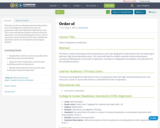Unrestricted Use
CC BY
Rating
0.0 stars

This lesson is about evaluating numerical expressions, and it was designed for adult learners who are preparing to take their High School Equivalency tests. This course will help the students evaluate numerical expressions correctly by following the correct order of operations, which includes the four basic arithmetical operations and the use of exponents and grouping symbols.

Subject:
Mathematics
Material Type:
Lesson
08/08/2019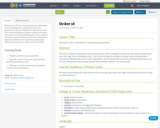Unrestricted Use
CC BY
Rating
0.0 stars

This lesson is about evaluating numerical expressions, and it was designed for adult learners who are preparing to take their High School Equivalency tests. This course will help the students evaluate numerical expressions correctly by following the correct order of operations, which includes the four basic arithmetical operations and the use of exponents and grouping symbols (parentheses, brackets, and curly braces).

Subject:
Mathematics
Material Type:
Lesson
08/08/2019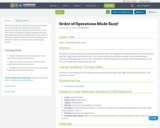Unrestricted Use
CC BY
Rating
0.0 stars

This lesson is about evaluating numerical expressions, and it was designed for adult learners who are preparing to take their High School Equivalency tests. This course will help the students evaluate numerical expressions correctly by following the correct order of operations, which includes the four basic arithmetical operations and the use of exponents and grouping symbols.

Subject:
Mathematics
Material Type:
Lesson